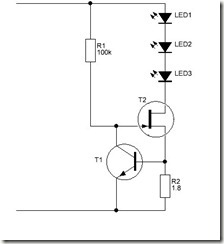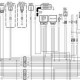# Power LED driversThe design of this LED driver is very simple and probably can be found in many variations on the interenet: T2 operates in linear mode and acts as a variable resistor that controls the current through the LED’s. R1 pulls-up the gate of T2 so that T2 starts up in its conductive state.
As the current begins to flow through the LED’s, T2, and R2, the voltage drop across R2 increases until it is high enough to switch on T1, and in so doing starts to pull the gate of T2 to ground.
This causes T2 to increase its resistance, which decreases current flow through itself, the LED’s, and R2, which decreases the voltage drop across R2, which causes T1 to let T2’s gate float back toward positive supply, thus increasing the current flow. Within a few milliseconds, the current flow stabilizes around a specific set point determined by the value of R2. The required supply voltage overhead is only about 0,6-1.2 volts, less than half of what for instance an LM317 regulator circuit requires.

As the value of R2 is based on the transition emitter-base voltage of T1, calculating it is easy. Most conventional silicon general-purpose NPN transistors switch fully at around 0.7VDC and start to transition from off to on at 0.56-0.58VDC. Assuming a transition voltage of 0.58VDC and a target load current of 320mA, the math is as follows (Ohms law):

R = V / I
R = 0.58VDC / 0.320A
R = 1.8 Ohms

Calculating the power dissipation for R2 under normal operation is done by Watt’s Law:

P = V * I
P = 0.58VDC * 0.320A
P = 0.185 Watt

In this case a quarter-watt resistor will work but a one-watt would have a safety margin of double. Using a design with an LM317 would lead to a much greater loss.

Choosing the input voltage is simple. In the example above I have used 3 LED’s, so you would have to total the forward voltage of those and add about 1.2 Volts:

For example:
Supply voltage for red LEDs=3*2.5V+1.2V=8.7V
Supply voltage for blue LEDs=3*3.8V+1.2V=12,6V

Perhaps you could even get away with an excess voltage of 0.6 instead of 1.2 Volts

The circuit has a disadvantage: It tries to push as much current through the load as it can as long as the voltage across R2 doesn’t exceed what starts to turn T1 on. So, for increased fault tolerance, the power rating for R2 could be selected based on the worst-case scenario of the maximum amount of available current flow in the event of a failure: a short across the LED’s as well as T2. For that, simply calculate dropping the supply power (voltage times current) across R1. It’s generally cheaper to simply use a fuse rated for just above the desired current set point, though, as a power supply providing 1A @ 12VDC would require a 15-watt resistor for R2 and this would be far more costly than a simple fuse.

Dissipation through the MOSFET under operating conditions is calculated the same way, only the voltage used in the calculation should be the supply voltage so that the heatsink selection will account for the worst-case scenario of a dead-shorted load at the target current. In the above example, assuming a 12VDC supply and 320mA current limit, the math works out as follows:

P = V * I
P = 12VDC * 0.750A
P = 3.84 watts

The IRF520 can handle 9.2A at 100VDC, and can dissipate 60 watts, so only a modest heatsink might be necessary.
To calculate dissipation through T2 with an accidentally shorted load, simply calculate the maximum supply power that T2 will see. If the supply is unregulated and capable of large current flows in short time spans (e.g., batteries), again a fuse would be wise as a last-ditch protective measure against catastrophic failures by blowing the circuit open so it cannot try to short the supply. If the set current is 320mA, a 500mA fuse in series with this circuit would be a wise, and relatively inexpensive, addition – all the more so for portable projects using lithium batteries, given how these like to catch fire (and for nonrechargeable lithium primaries, explode) when shorted.
The only limiting factors on load current and supply voltage relate to the components – using a suitable resistor for R2, this circuit can easily regulate up to a few amps of current in as little as one square inch of board space. R1 is not too critical: 1Megaohm will work and I also have seen 10k for R1 and 33Ohm for R2 driving 3 LED’s at a 12 V supply. A good way to calculate the value of R1 is: R1= (Vcc – 1.4)/(I/20)

If you need precision, the easiest way to get it is to build a test circuit with a fixed supply (12VDC is a great value, but use the desired or intended supply voltage if there’s a need to be precise), a dead short for a load, and a 100 ohm resistor for R2. This will set the current limit to about 6mA. Once the circuit is powered up, measure the drop across R2 to determine the exact transition voltage for T2, and calculate a specific value for R2 for your current requirements based on the math described earlier.

PWM
The extra parts for PWM support include T3, R3. Since T3 is a PNP transistor and R4 biases its base to ground, T3 starts up turned on and pulls T1’s gate to ground regardless of what T2 is doing, and this forces both T1 and T2 to turn off. R3 limits current draw to the PWM signal source. Provide a positive voltage greater than ~0.7VDC to T3 through R3, and T3 turns off, which allows the rest of the regulator to function as described above. In this manner a PWM signal can be used to vary the brightness of a load of power LEDs, or a simple on-off switch effect can be implemented by merely pulling T3’s base to positive supply with a few milliamperes of current. In this manner it would be possible to use the circuit as a self-current-limiting switch that only needs a tiny little low-current button as its actuator even though the load could be an amp or more.

For LEDs, this circuit is  very simple and wastes very little power if the source and load voltages are kept close to each other. Ideally this circuit works most efficiently with the supply being right at 1.5VDC above the ideal load voltage at the set current. The power source should be able to supply the required amount of current.

If the circuit is used for other loads than LED’s there will be some ripple depending on the type of load being powered, especially during power-up, but decoupling capacitors could be used to compensate for this. The circuit can be driven directly from any logic circuit (even at 3.3VDC).

The circuit can drive resistive, inductive, capacitive, or mixed loads. Capacitive loads may well experience slower charge times, as the circuit will clamp the charge current. Inductive loads should be paralleled with a reverse-polarity diode as is common with e.g. relais (and in some cases, also a proper snubber capacitor) to suppress any back-EMF from the load when power is disconnected so that the voltage spike won’t damage the MOSFET. (Most power MOSFETs include integrated protection diodes, but this should never be relied upon as the sole protection mechanism.)

Even simpler
If you do not have a MOSFET, but you happen to maybe still have an old trusty 2N3055 (or similar like the TIP series) workhorse laying around, the following circuit is for you:

The current is determined by R2 and is:
I =0.8/R2

The maximum value of I in this driver is 10A. .
DC-power supply 9V-15V DC

R2 for 1W LED -2.7ohms 1W
R2 for 3W LED -1.5-ohms 1W
R2 for 5W LED -0.6-ohms or 2 x 1.2-ohms/1W in parallel

Using an LEDInstead of 2 diodes it is also possible to use an LED to set the reference voltage. Ofcourse for this one needs to know the exact voltage of the LED.
Calculations of the resistors is as follows:
R1=(Vd-Vbe)/IR1
R2=(Vs-Vd)/(Id*KB) in which Vd= the LED voltage, Id the current through the circuit LED and where K = 1.2 to 2 (so that R1 is low enough to ensure adequate IB)

## 7 thoughts on “Power LED drivers”

1.matt2005 says:

Reblogged this on matt2005.

2.Arduino says:

Matt, Thanks for letting me know

3.ohms law says:

If some one wants to be updated with newest technologies then he must be pay a
quick visit this website and be up to date daily.

4.Pablo Matias says:

hello, I want to connect 2 led 1500mH 3.1v in 12v and control the PWM with Arduino, how should I proceed?

1.Arduino says:

Pablo apologies for not replying to you sooner but I overlooked your question.
with 1500mH i presume you mean “1500mA”
Well there are several ways you can do that. You could do that with a Single Fet + a few resistors
1: get an N-logic FET such as the STP20NF06L
2: connect the source to ground.
3: attach the gate to yr Arduino via a 1k resistor
4: Attach the gate to ground via a 220 k resistor
5: in the drainline you have to put a resistor and your LED’s all in series and then connect that to your 12 Volt
6: as the fall over the FET is smallyou would need to let (12-2×3.1=)5.8 Volts fall over the resistor at 1500 mA -> R=3.9 Ohm. 5.8×1.5=8.7 Watt
7: send PWM signal to the resistor on the gate

As an 8.7 Wat resistor is a bit Bulky and pricy it is better to use the driver as pictured under the caption ‘PWM’ in my article. The only thing you need to do is to calculate the setting resistor. This is about 0.5 Ohm / 750 mW (take a 1 Watt).

I must say though that feeding 2 3.1 Volt LED’s with 12 Volt at 1.5 Amps is wasting a lot of energy. The LED will use 4.65 Watt, but the circuit will use an extra 8.7 Watt on top of that so that is only an efficiency of (4.65/(4.65+8.7))*100= 35% –> 65% of your energy will be turned into heat immediately and that isnt even counting the heat produced by the LED

5.V4n14 says:

Dear Arduino, I had 4 strips of LED back light TV, which 75 Volt each, I made series connection become 300V(supply direct rectified 220V line), total 28Watt.
I had also RGB remote dimmer controller, 24V, 72Watt max, I checked inside the output is 3 pcs of SMD Mosfet nChannel (written A2) common Anode , how is the best interfacing them ?, I like your diagram no.2 above about PWM, is it possible to replace the mosfet with IRF740 ?, Is it save for PWM circuit ?, or we need an opto coupler 4N35 ?, thanks for your spending time.

1.E says:

Hard to say how to best interface them as i do not know the circuit of your remote dimmer. I presume that with common anode you mean that yoyr RGB LED is common anode. The circuit nr 2 is indded suitable for common anode and I see no reason why you couldnt use the IRF740.

But I would strongly advise to use an optocoupler if you plan to connect anything directly to the mains.

With regard to your dimmer… in theory if you would replace your smd FETs with higher power FETs that should work…. in theory

This site uses Akismet to reduce spam. Learn how your comment data is processed.